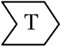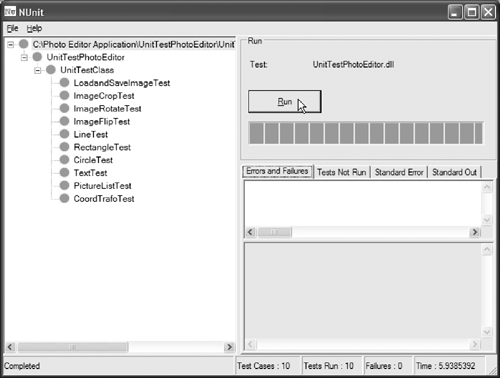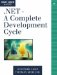# Unit TestsThe unit tests for this iteration are added to the unit tests developed in Chapter 5. The strategy is to test the units and, in particular, to test units that are used often and that are used by many components. For that reason, in addition to the tests of the line, circle, rectangle, and text components we develop tests for the coordinate transformation and picking functionalities.

The tests for the graphics components basically check whether the coordinates of the start and end points are stored and retrieved correctly. In addition, we test the picking of the components, the coordinate transformation, and the list that holds the components.

As a starting point, Listing 6.12 shows the rectangle unit test, which sets the start and end points. The points are set, and a check is implemented to verify that the coordinates correspond to the original coordinates after they are retrieved. In addition, we check whether picking works correctly on the component.

Next, the component list test is shown. The test creates several components, puts them into a list, and checks whether they are still the same. To support the testing, we put a hook into the Picture component. This hook is an accessor method called GraphicsComponentList, and it enables us to retrieve a reference to the component list of the PictureComponent class (obviously, the hook is implemented in the PictureComponent class). The test also removes entries from the list and checks whether the correct entries were removed by checking the removed components against the original list and by comparing the count of the list members with an expected value. In addition, the list is emptied and we check whether the list does not contain any objects after it was cleared.

The final test case checks whether the calculated viewport coordinates are correct. This is done by setting artificial viewport coordinates that are used to calculate expected values when the viewport is not set to the origin (meaning that the image has been scrolled).

Listing 6.12 Unit Tests for GDI+ Components

`/// `

/// Test for F:image_graphics_annotations. /// Sets the start and end points and /// checks whether they were set correctly. /// Also checks whether picking is done /// correctly. ///

/// F:image_graphics_annotations [Test] public void RectangleTest() { Console.WriteLine("image_graphics_annotations"); RectangleComponent myRect = new RectangleComponent(); myRect.StartPoint = new Point(0, 0); myRect.EndPoint = new Point(100, 200); Assertion.AssertEquals("Rectangle (0, 0) (100, 200)", myRect.StartPoint.X, 0); Assertion.AssertEquals("Rectangle (0, 0) (100, 200)", myRect.StartPoint.Y, 0); Assertion.AssertEquals("Rectangle (0, 0) (100, 200)", myRect.EndPoint.X, 100); Assertion.AssertEquals("Rectangle (0, 0) (100, 200)", myRect.EndPoint.Y, 200); Assertion.AssertEquals("Pick rectangle test", myRect.IsPicked(0, 0), true); Assertion.AssertEquals("Pick rectangle test", myRect.IsPicked(97, 198), true); Assertion.AssertEquals("Pick rectangle test", myRect.IsPicked(95, 200), false); myRect.StartPoint = new Point(200, 10); myRect.EndPoint = new Point(10, 5); Assertion.AssertEquals("Rectangle (200, 10) (10, 5)", myRect.StartPoint.X, 200); Assertion.AssertEquals("Rectangle (200, 10) (10, 5)", myRect.StartPoint.Y, 10); Assertion.AssertEquals("Rectangle (200, 10) (10, 5)", myRect.EndPoint.X, 10); Assertion.AssertEquals("Rectangle (200, 10) (10, 5)", myRect.EndPoint.Y, 5); Assertion.AssertEquals("Pick rectangle test", myRect.IsPicked(197, 12), true); Assertion.AssertEquals("Pick rectangle test", myRect.IsPicked(13, 7), true); Assertion.AssertEquals("Pick rectangle test", myRect.IsPicked(0, 10), false); amIPassed = true; } ///

/// Test for F:image_graphics_annotations. /// Creates components, puts them in the list, and /// removes them to check whether the list is handled /// correctly. ///

/// F:image_graphics_annotations /// F:image_text_annotations [Test] public void PictureListTest() { Console.WriteLine("image_graphics_annotations"); int i; ArrayList lineList = new ArrayList(10); for(i = 0; i < 10; ++i) { lineList.Add(new LineComponent()); TestImage.Add((LineComponent)lineList[i]); } ArrayList cList = TestImage.ComponentList; for(i = 0; i < 10; ++i) { Assertion.AssertEquals("Line list test ", lineList[i], cList[i]); Assertion.AssertEquals("Line list test ", lineList.Count, 10); } TestImage.Remove((Component)lineList); lineList.Remove(lineList); for(i = 0; i < 9; ++i) { Assertion.AssertEquals("Line list test ", lineList[i], cList[i]); Assertion.AssertEquals("Line list test ", lineList.Count, 9); } TestImage.Remove((Component)lineList); lineList.Remove(lineList); for(i = 0; i < 8; ++i) { Assertion.AssertEquals("Line list test ", lineList[i], cList[i]); Assertion.AssertEquals("Line list test ", lineList.Count, 8); } Assertion.AssertEquals("Line list test ", lineList.Count, cList.Count); lineList.Clear(); cList.Clear(); Assertion.AssertEquals("Line list test ", lineList.Count, cList.Count); amIPassed = true; } ///

/// Test for F:image_graphics_annotations. /// Sets the start point and /// checks the coordinate /// transformation. ///

/// F:image_graphics_annotations [Test] public void CoordTrafoTest() { Console.WriteLine("image_graphics_annotations"); RectangleComponent myRect = new RectangleComponent(); myRect.StartPoint = new Point(0, 0); myRect.ViewportCoords = new Point(10, 500); Assertion.AssertEquals("Rectangle Viewport Coords (0, 0) => (10, 500)", myRect.CalcViewportCoords(myRect.StartPoint).X, -10); Assertion.AssertEquals("Rectangle Viewport Coords (0,0) => (10, 500)", myRect.CalcViewportCoords(myRect.StartPoint).Y, - 500); myRect.StartPoint = new Point(200, 651); Assertion.AssertEquals("Rectangle Viewport Coords (200, 651) => (10, 500)", myRect.CalcViewportCoords(myRect.StartPoint).X, 190); Assertion.AssertEquals("Rectangle Viewport Coords (200, 651) => (10, 500)", myRect.CalcViewportCoords(myRect.StartPoint).Y, 151); amIPassed = true; }

## Do It Yourself

Add similar tests for the line, circle, and text components. A sample solution is provided on the CD. Also think of more and better unit test cases. The provided cases can be seen as a very minimal set of tests. You are encouraged to cook up more tests to find hidden problems in the software.

Figure 6.13 shows the output of the sample unit test.

Figure 6.13. Unit Test Output.NET-A Complete Development Cycle
ISBN: 0321168828
EAN: 2147483647
Year: 2005
Pages: 123